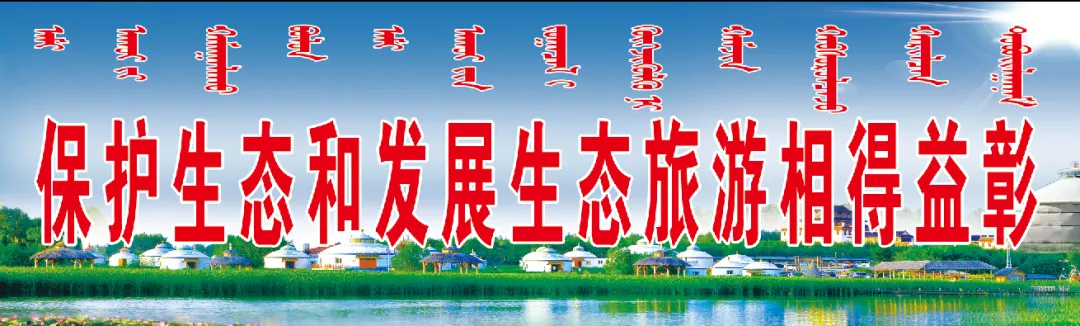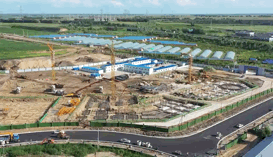•客户端下载

##### 【通告】关于查干湖生态小镇项目房屋征收与补偿决定的通告1.查干湖生态小镇项目整体规划范围内坐标。

x=5017588.159/y=385217.508至坐标x=5017545.973/y=385352.420x=5017534.010/y=385358.667x=5017423.318/

y=385318.401—x=5017417.278/y=385305.563—x=5017462.769/y=385177.922—x=5017475.666/y=385171.500—x=50

17581.669/y=385204.808—x=5017588.159/y=385217.508范围内的房屋及附属物和土地。

2.查干湖生态小镇项目整体规划范围内坐标。

x=5017328.772/y=385476.545至坐标x=5017185.408/y=385408.579—x=5017145.750/y=385340.545—x=5017124.770/y=385297.355—x=5016983.406/y=385226.453—x=5016978.526/y=385213.179—x=5017429.837/y=384630.948—x=5017447.637/y=384627.040—x=5017494.342/y=384679.421—x=5017444.059/y=384730.479—x=5017444.290/y=384912.127—x=5017404.013/y=384916.136—x=5017394.440/y=385049.069—x=5017308.843/y=385333.785—x=5017397.407/y=385364.164—x=5017328.772/y=385476.545范围内的房屋及附属物和土地。

2020年12月15日